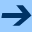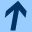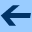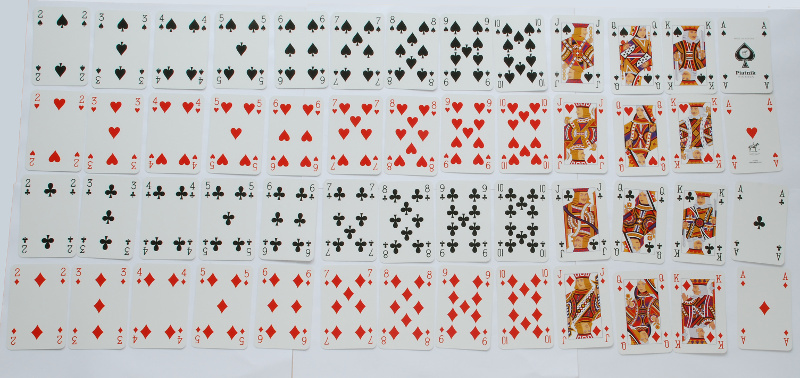More about classes

# More about classes

So far all our classes where data classes, because the compiler provides some nice support for these: a reasonable toString method, equality testing, and they can be used as elements in a set or as keys in a map.

Data classes are meant for objects that have a number of basic attributes, like numbers and strings, and there are some restrictions on their definition. In this section we will work with general classes—we will review the differences at the end.

#### Constructor arguments

When creating objects, we are often providing arguments for the constructor, as for instance when we made a Date:

  >>> var d = Date(2012, 4, 9)


The arguments 2012, 4, 9 correspond to the parameters year, month, and day that appear in parentheses after the class name in the class declaration. The constructor parameters can be val fields, var fields, or simply parameters for the construction of the class.

Here is an immutable Point class. Both class parameters are val fields:

class Point(val x: Int, val y: Int) {
override fun toString(): String = "(%d, %d)".format(x, y)
}

Here is a mutable Rect class to store an axis-parallel rectangle. The class parameters are var fields:
class Rect(var corner: Point, var width: Int, var height: Int) {
init { require(width > 0 && height > 0) }
override fun toString(): String = "[%d ~ %d, %d ~ %d]".format(corner.x,
corner.x + width, corner.y, corner.y + height)
}


The first class parameter is a Point object, so I should create rectangle objects like this:

>>> var r = Rect(Point(10, 20), 50, 20)
>>> r
[10 ~ 60, 20 ~ 40]


Let's say I don't like this, I want to be able to create rectangles by giving four numbers, like this:

>>> var r = Rect(10, 20, 50, 20)
>>> r
[10 ~ 60, 20 ~ 40]


I still want to be able to store the top-left corner as a Point object, but because the class parameters now look different, I cannot define the corner field in the class parameter list. Instead I need to do it like this:

class Rect(x: Int, y: Int, var width: Int, var height: Int) {
var corner = Point(x, y)
init { require(width > 0 && height > 0) }
override fun toString(): String = "[%d ~ %d, %d ~ %d]".format(corner.x,
corner.x + width, corner.y, corner.y + height)
}


Now the corner field is defined in the body of the class. The class parameters x and y are simply parameters of the method that constructs objects of type Rect, they are not fields of the class:

>>> r.corner
(10, 20)
>>> r.width
50
>>> r.height
20
>>> r.x
error: unresolved reference: x
>>> r.y
error: unresolved reference: y


So every occurrence of val or var in the body of the class defines a field of the class. Every field has to be initialized with a starting value. This value is used when the object is constructed.

#### Privacy

Let us define an Accumulator, a counter that starts with zero, and to which we can add a value (accum1.kts):

class Accumulator {
var sum = 0
fun add(n: Int) {
sum += n
}
}

The class itself has no class parameters this time, so no arguments are given when we create Accumulator objects:
>>> var acc1 = Accumulator()
>>> acc1
Line46$Accumulator@747f281 >>> acc1.add(7) >>> acc1.add(13) >>> acc1.sum 20  (Note that since Accumulator is not a data class, it has a rather ugly toString method by default. We would have to override toString to get nicer output.) What is not so nice here is that we could accidentally modify the sum-field of the Accumulator object: >>> var acc2 = Accumulator() >>> acc2.add(7) >>> acc2.add(23) >>> acc2.sum = 0 // Oops >>> acc2.add(19) >>> acc2.sum 19  The programmer using the Accumulator class made a mistake here and set acc2.sum back to zero—so now the final result is wrong. This is an example that shows the importance of privacy. A client—that is, code using our class— should consider an object as a black box. The client should not need or want to know how the object is implemented, and must only use the methods provided by the object to communicate with it. Our Accumulator object should have two operations: adding a number to the current sum, and reading out the current sum. It should not be possible to modify the current sum. We can achieve this by forbidding client code to access the field that stores the current sum. To do so, we declare the field to be private. However, that means that we cannot access it at all, so we have to add a new method to be able to read the current value of the summation (accum2.kts): class Accumulator { private var current = 0 fun add(n: Int) { current += n } fun sum(): Int = current }  Here is how we use it correctly: >>> var acc1 = Accumulator() >>> acc1.add(7) >>> acc1.add(13) >>> acc1.sum() 20  But now see what happens when we make a mistake and try to change the sum: >>> acc1.sum = 0 error: function invocation 'sum()' expected error: variable expected  Since sum is not a field but a method that returns a value, we cannot assign to it. And even if we tried to change the field current directly, the compiler would catch this programming error: >>> acc1.current = 0 error: cannot access 'current': it is 'private' in 'Accumulator'  In fact, even looking at the value of current is forbidden (that's why we needed the sum method): >>> acc1.current error: cannot access 'current': it is 'private' in 'Accumulator'  The private keyword means that the member can be accessed only from methods inside the class. You can use it both for fields and for methods. So a private method is a method that can be called only from other methods in the same class. #### A Blackjack game To see a more interesting example, let us program a Blackjack game. We first need a class that represents the playing cards. Remember that there are four suits called clubs, spades, hearts, and diamonds, and 13 faces, namely 2 to 10, Jack, Queen, King, and Ace.Since this is a simple class, we should make it a data class. We override toString anyway to obtain a nice string representation (blackjack1.kt): val Suits = arrayOf("Clubs", "Spades", "Hearts", "Diamonds") val Faces = arrayOf("2", "3", "4", "5", "6", "7", "8", "9", "10", "Jack", "Queen", "King", "Ace") data class Card(val face: String, val suit: String) { init { require(suit in Suits) require(face in Faces) } override fun toString(): String { val a = if (face == "Ace" || face == "8") "an " else "a " return a + face + " of " + suit } fun value(): Int = when(face) { "Ace" -> 11 "Jack" -> 10 "Queen" -> 10 "King" -> 10 else -> face.toInt() } }  Note the value method, which returns the number of points that the card is worth. >>> val c1 = Card("Ace", "Diamonds") >>> val c2 = Card("Jack", "Spades") >>> val c3 = Card("8", "Hearts") >>> c1 an Ace of Diamonds >>> c2 a Jack of Spades >>> c3 an 8 of Hearts >>> val hand = listOf(c1, c2, c3) >>> hand [an Ace of Diamonds, a Jack of Spades, an 8 of Hearts] >>> for (c in hand) ... println("Card$c has value ${c.value()}") Card an Ace of Diamonds has value 11 Card a Jack of Spades has value 10 Card an 8 of Hearts has value 8  We next need a class Deck that stores an entire deck of cards, and allows us to draw cards from the deck (blackjack2.kt): class Deck { private val cards = mutableListOf<Card>() init { generateDeck() shuffleDeck() } private fun generateDeck() { for (suit in Suits) { for (face in Faces) { cards.add(Card(face, suit)) } } } private fun shuffleDeck() { for (i in 1 .. 52) { // 0..i-2 already shuffled val j = random.nextInt(i) val cj = cards[j] cards[j] = cards[i-1] cards[i-1] = cj } } fun draw(): Card { assert(!cards.isEmpty()) return cards.removeAt(cards.lastIndex) } }  Note that Deck has no class parameter, so it is created simply by saying Deck() without any arguments. Let's look at the first lines of the class more carefully:  private val cards = mutableListOf<Card>() init { generateDeck() shuffleDeck() }  The first line defines a val-field named cards. This field is initialized with an empty mutable list. So before the Deck object can be used, this list has to be filled with the actual cards. This is done by the two method calls generateDeck() and shuffleDeck(). Both are private methods of Deck: That is, they are methods of Deck, but they cannot be called directly by client code. They are purely for internal use of the class (in our case for use in the constructor, to set up a properly shuffled deck of the 52 Blackjack cards). The first method generateDeck fills the array with the 52 cards, the second method shuffleDeck rearranges them into a random order. Finally, the draw method is not private: It is meant for clients of the Deck to draw one card from the deck. Here is an example of using the Deck class: >>> val deck = Deck() >>> for (i in 1 .. 10) ... println(deck.draw()) a King of Spades a 3 of Diamonds a Jack of Hearts a 7 of Hearts a 10 of Diamonds an Ace of Spades a Queen of Clubs a 4 of Clubs a 2 of Clubs a Jack of Clubs  Now that we have a working deck, we can write the client code, that is, we can implement the complete Blackjack game. Here is the main function that implements one game (you can download the entire program as blackjack-game.kt). Note how easy it is to read this code, because we have hidden all the complexity of storing cards and shuffling and managing the deck inside the Card and Deck classes. // Play one round of Blackjack // Returns 1 if player wins, -1 if dealer wins, and 0 for a tie. fun blackjack(): Int { val deck = Deck() // initial cards var player = mutableListOf(deck.draw()) println("You are dealt " + player.first()) var dealer = mutableListOf(deck.draw()) println("Dealer is dealt a hidden card") player.add(deck.draw()) println("You are dealt " + player.last()) dealer.add(deck.draw()) println("Dealer is dealt " + dealer.last()) println("Your total is${handValue(player)}")

// player's turn to draw cards
var want = true
while (want && handValue(player) < 21) {
want = askYesNo("Would you like another card? (y/n) ")
if (want) {
player.add(deck.draw())
println("You are dealt " + player.last())
println("Your total is ${handValue(player)}") // if the player's score is over 21, the player loses immediately. if (handValue(player) > 21) { println("You went over 21! You lost!") return -1 } } } println("The dealer's hidden card was " + dealer.first()) while (handValue(dealer) < 17) { dealer.add(deck.draw()) println("Dealer is dealt " + dealer.last()) } println("The dealer's total is${handValue(dealer)}")

// summary
val player_total = handValue(player)
val dealer_total = handValue(dealer)
println("\nYour total is $player_total") println("The dealer's total is$dealer_total")

if (dealer_total > 21) {
println("The dealer went over 21! You win!")
return 1
} else if (player_total > dealer_total) {
println("You win!")
return 1
} else if (player_total < dealer_total) {
println("You lost!")
return -1
} else {
println("You have a tie!")
return 0
}
}


To compile the game, we need the Date and Deck classes (from blackjack2.kt) and the game functions (from blackjack-game.kt), so we can compile like this:

$ktc blackjack2.kt blackjack-game.kt  We run the game through the main function in blackjack-game.kt, that is like this: $ kt Blackjack_gameKt
Welcome to Blackjack!

You are dealt an 8 of Spades
Dealer is dealt a hidden card
You are dealt a 5 of Clubs
Dealer is dealt a Queen of Hearts
Your total is 13
Would you like another card? (y/n) y
You are dealt a 9 of Diamonds
Your total is 22
You went over 21! You lost!

Play another round? (y/n) n


#### So what about data classes?

In a previous chapter we have learnt about data classes. In this chapter we have started to talk about normal classes. So what's the difference?

The short answer is: Data classes are for small objects that have no hidden state. Our Deck class was an example of an object with hidden state, namely the current cards on the deck.

The long answer is that data classes are different from normal classes in the following ways:

• For normal classes, the toString method just prints the class name and some string in hexadecimal. For data classes, it prints the class name and the values of all the fields. If we want a pretty toString method for normal classes, we need to override the toString method and define it ourselves.
• For normal classes, the equality operators == and != only test if the two references refer to the same object. For data classes, two objects are equal if all their fields are equal:
>>> class Normal(val x: Int)
>>> val n1 = Normal(7)
>>> val n2 = Normal(7)
>>> val n3 = n1
>>> n1 == n2
false
>>> n1 == n3
true
>>> n2 == n3
false
>>> data class Data(val x: Int)
>>> val d1 = Data(7)
>>> val d2 = Data(7)
>>> val d3 = d1
>>> d1 == d2
true
>>> d1 == d3
true
>>> d2 == d3
true

If you want to redefine the meaning of == and != for normal classes, you have to define an equals method.
• Immutable data class objects can be used as elements of a set or as keys of a map. For normal classes, this will only work if you define equals and hashCode, but that goes beyond the scope of this tutorial.
• Multiple data class fields can be extracted in one step:
>>> data class Date(val year: Int, val month: Int, val day: Int)
>>> val d = Date(2012, 9, 12)
>>> d
Date(year=2012, month=9, day=12)
>>> fun extract(date: Date) {
...   val (y, m, d) = date
...   println("$y/$m/\$d")
... }
>>> extract(d)
2012/9/12

The assignment val (y, m, d) = date works because date is a data class object. For normal classes, you would have to define the componentX methods.
• Data classes automatically have a copy method that allows you to make copies where you selectively change some field, for instance like this:
>>> d
Date(year=2012, month=9, day=12)
>>> val d1 = d.copy(month = 12)
>>> d1
Date(year=2012, month=12, day=12)

For normal classes you would have to define such a method by hand.More about classes